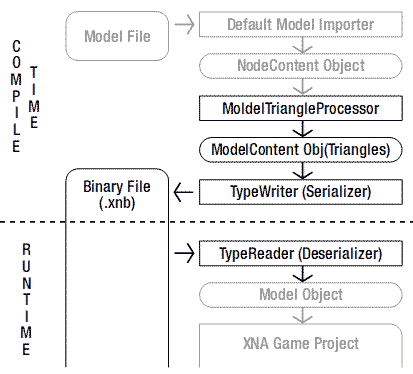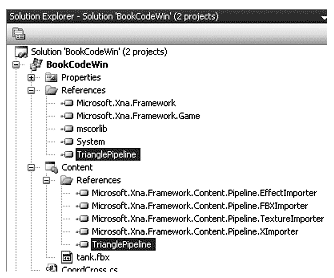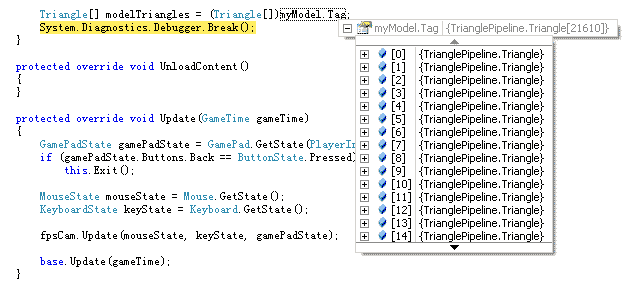## 工作原理public class Triangle
{
private Vector3[] points;

public Triangle(Vector3 p0, Vector3 p1, Vector3 p2)
{
points = new Vector3;
points = p0;
points = p1;
points = p2;
}

public Vector3[] Points { get { return points; } }
public Vector3 P0 { get { return points; } }
public Vector3 P1 { get { return points; } }
public Vector3 P2 { get { return points; } }
} 

Process类的代码几乎和教程4-13中的一样，但这次你保存的是Triangle对象的集合而不是上一个教程的Vector3的集合。在Triangle类之后添加Processor类：

[ContentProcessor]
public class ModelTriangleProcessor : ModelProcessor
{
public override ModelContent Process(NodeContent input, ContentProcessorContext	context)
{
ModelContent usualModel = base.Process(input, context);

List<Triangle> triangles = new List<Triangle>();

usualModel.Tag = triangles.ToArray();
return usualModel;
}
} 

private List<Triangle> AddVerticesToList(NodeContent node, List<Triangle>triangleList)
{
MeshContent mesh = node as MeshContent;

if (mesh != null)
{
Matrix absTransform = mesh.AbsoluteTransform;

foreach (GeometryContent geo in mesh.Geometry)
{
//generate Triangle objects...
}
}

foreach (NodeContent child in node.Children)

return triangleList;
} 

int triangles = geo.Indices.Count / 3;
for (int currentTriangle = 0; currentTriangle < triangles; currentTriangle++)
{
int index0 = geo.Indices[currentTriangle * 3 + 0];
int index1 = geo.Indices[currentTriangle * 3 + 1];
int index2 = geo.Indices[currentTriangle * 3 + 2];

Vector3 v0 = geo.Vertices.Positions[index0];
Vector3 v1 = geo.Vertices.Positions[index1];
Vector3 v2 = geo.Vertices.Positions[index2];

Vector3 transv0 = Vector3.Transform(v0, absTransform);
Vector3 transv1 = Vector3.Transform(v1, absTransform);
Vector3 transv2 = Vector3.Transform(v2, absTransform);

Triangle newTriangle = new Triangle(transv0, transv1, transv2);
} 

Unsupported type. Cannot find a ContentTypeWriter implementation for ModelTriaPipeline.Triangle

### 编写自定义内容TypeWriter

[ContentTypeWriter]
public class TriangleTypeWriter : ContentTypeWriter<Triangle>
{
protected override void Write(ContentWriter output, Triangle value)
{
output.WriteObject<Vector3>(value.P0);
output.WriteObject<Vector3>(value.P1);
output.WriteObject<Vector3>(value.P2);
}

{
}
} 

public class TriangleTypeReader : ContentTypeReader<Triangle>
{
{

Triangle newTriangle = new Triangle(p0, p1, p2);
return newTriangle;
}
} 

### 在内容管道项目中添加引用

Cannot find ContentTypeReader ModelTriaPipeline.Triangle, ModelTriaPipeline,
Version=1.0.0.0, Culture=neutral### 添加内容管道的命名空间

ModelTriaPipeline.Triangle[] modelTriangles = (ModelTriaPipeline.Triangle[])myModel.Tag;

using ModelTriaPipeline;

Triangle[] modelTriangles = (Triangle[])myModel.Tag;

### 使用自定义类对象扩展处理器的步骤清单

1．在解决方案中添加一个内容管道项目

2．在新项目中，添加Microsoft. XNA. Framework. Content. Pipeline的引用。

3．在using代码块添加Pipeline命名空间。

4．表明你将扩展哪个部分(这部分的方法需要重写)。

5．编译新内容管道项目。

6．在主项目中添加新创建的引用

7．选择新创建的处理器处理一个素材。

8．设置项目依赖项。

9．在XNA主程序中，添加自定义内容管道的引用。

10．在XNA主程序中的using代码块中添加内容管道命名空间。

1．定义自定义类

2．编写第4步中的代码

3．对每个处理器中使用的自定义类，创建一个TypeWriter。

## 代码

namespace TrianglePipeline
{
public class Triangle
{
private Vector3[] points;
public Triangle(Vector3 p0, Vector3 p1, Vector3 p2)
{
points = new Vector3;

points = p0;
points = p1;
points = p2;
}

public Vector3[] Points { get { return points; } }
public Vector3 P0 { get { return points; } }
public Vector3 P1 { get { return points; } }
public Vector3 P2 { get { return points; } }
}

[ContentProcessor]
public class ModelTriangleProcessor : ModelProcessor
{
public override ModelContent Process(NodeContent input, ContentProcessorContext context)
{
ModelContent usualModel = base.Process(input, context);

List<Triangle> triangles = new List<Triangle>();

usualModel.Tag = triangles.ToArray();
return usualModel;
}

private List<Triangle> AddVerticesToList(NodeContent node, List<Triangle> triangleList)
{
MeshContent mesh = node as MeshContent;

if (mesh != null)
{
Matrix absTransform = mesh.AbsoluteTransform;
foreach (GeometryContent geo in mesh.Geometry)
{
int triangles = geo.Indices.Count / 3;
for (int currentTriangle = 0; currentTriangle < triangles; currentTriangle++)
{
int index0 = geo.Indices[currentTriangle *3+ 0];
int index1 = geo.Indices[currentTriangle *3+ 1];
int index2 = geo.Indices[currentTriangle *3+ 2];

Vector3 v0 = geo.Vertices.Positions[index0];
Vector3 v1 = geo.Vertices.Positions[index1];
Vector3 v2 = geo.Vertices.Positions[index2];

Vector3 transv0 = Vector3.Transform(v0, absTransform);
Vector3 transv1 = Vector3.Transform(v1, absTransform);
Vector3 transv2 = Vector3.Transform(v2, absTransform);

Triangle newTriangle = new Triangle(transv0, transv1, transv2);
}
}
}

foreach (NodeContent child in 	node.Children)
return triangleList;
}
}

[ContentTypeWriter]
public class TriangleTypeWriter : ContentTypeWriter<Triangle>
{
protected override void Write(ContentWriter output, Triangle value)
{
output.WriteObject<Vector3>(value.P0);
output.WriteObject<Vector3>(value.P1);
output.WriteObject<Vector3>(value.P2);
}

{
}
}

{
{

Triangle newTriangle = new Triangle(p0, p1, p2);
return newTriangle;
}
}
} 

myModel = Content.Load<Model>("tank");
modelTransforms = new Matrix[myModel.Bones.Count];

Triangle[] modelTriangles = (Triangle[])myModel.Tag;
System.Diagnostics.Debugger.Break();2006 - 2023，推荐分辨率1024*768以上，推荐浏览器Chrome、Edge等现代浏览器，截止2021年12月5日的访问次数：1872万9823 站长邮箱